Anzeige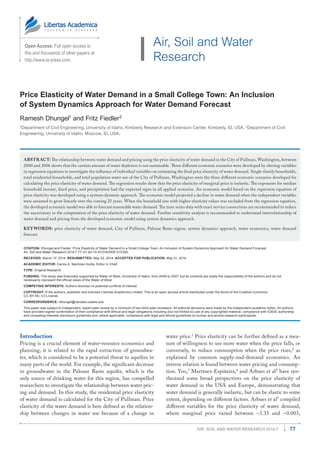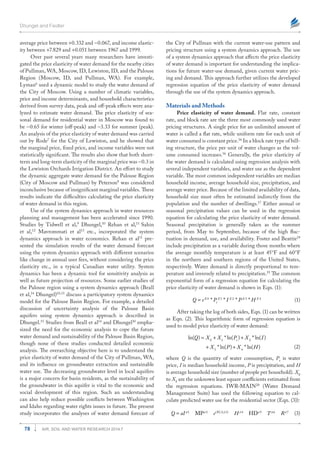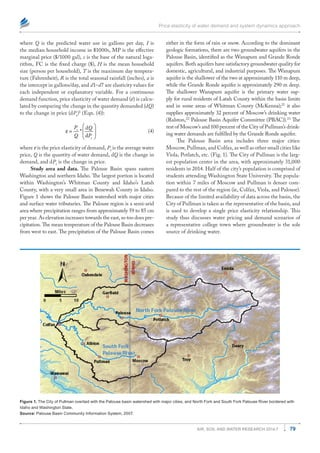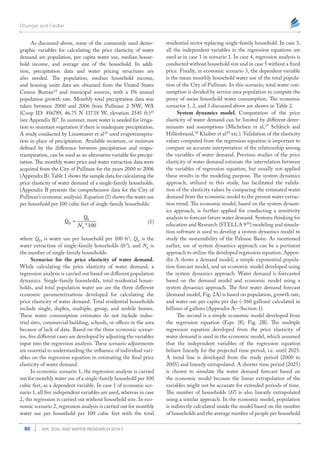Anzeige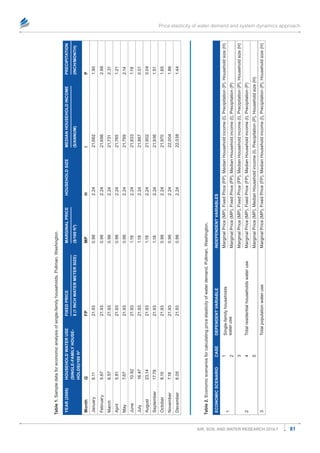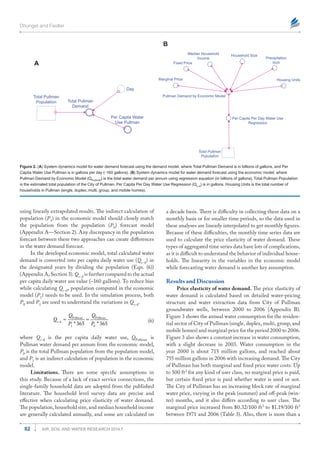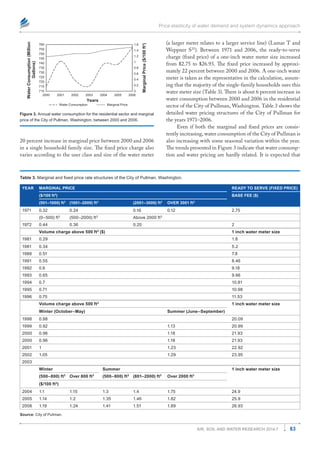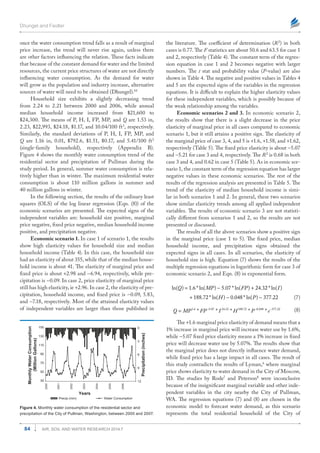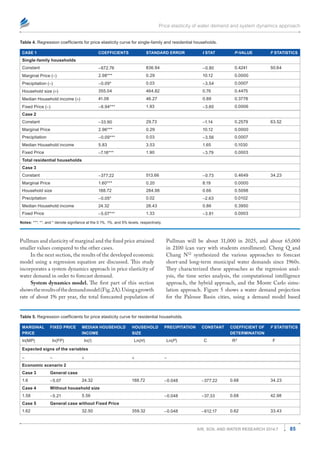Anzeige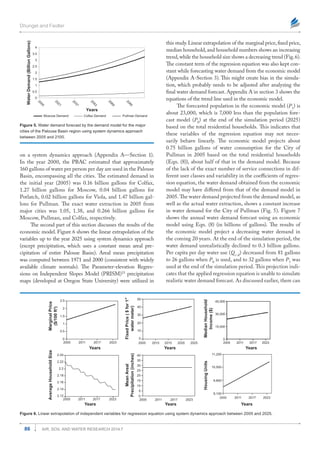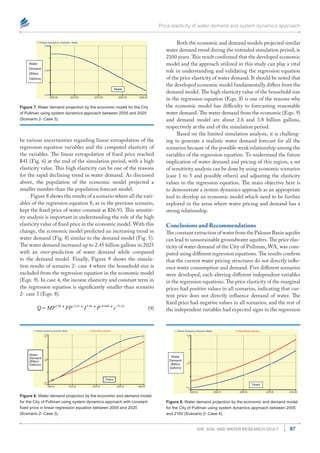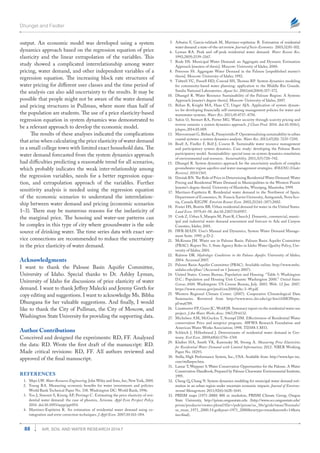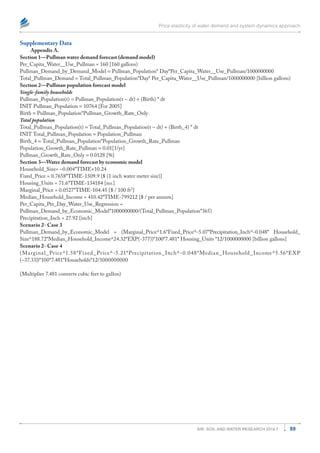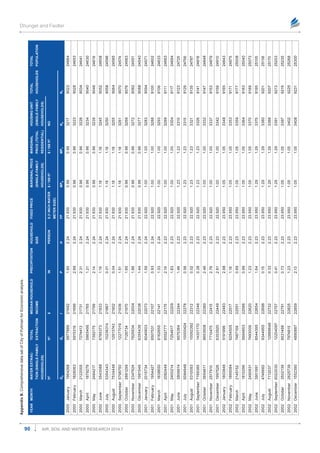Anzeige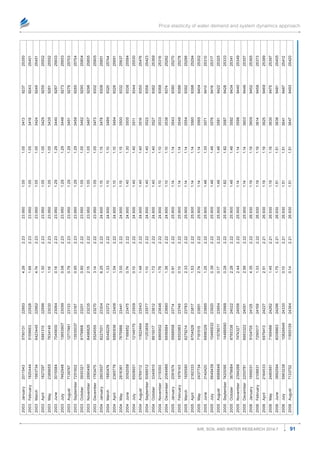Nächste SlideShare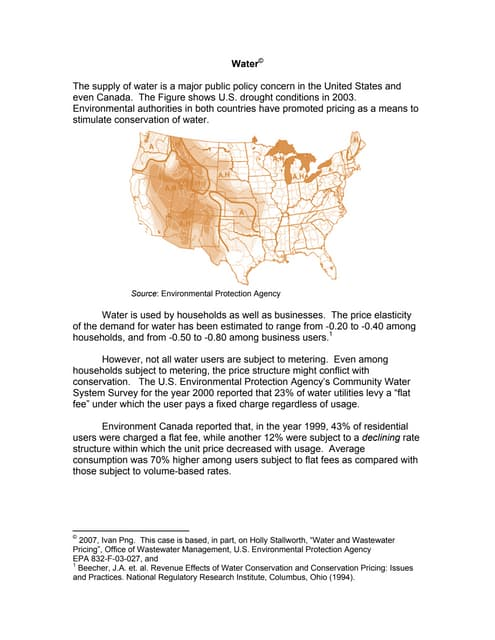Water case
1 von 15
Anzeige

### Price Elasticity of Water Demand in a Small College Town: An Inclusion of System Dynamics Approach for Water Demand Forecast

2. Dhungel and Fiedler 78 Air, Soil and Water Research 2014:7 ­average price between +0.332 and -0.067, and income elastic- ity between +7.829 and +0.051 between 1967 and 1999. Over past several years many researchers have investi- gated the price elasticity of water demand for the nearby cities of Pullman, WA, Moscow, ID, Lewiston, ID, and the Palouse Region (Moscow, ID, and Pullman, WA). For example, Lyman6 used a dynamic model to study the water demand of the City of Moscow. Using a number of climatic variables, price and income determinants, and household characteristics derived from survey data, peak and off-peak effects were ana- lyzed to estimate water demand. The price elasticity of sea- sonal demand for residential water in Moscow was found to be -0.65 for winter (off-peak) and -3.33 for summer (peak). An analysis of the price elasticity of water demand was carried out by Rode7 for the City of Lewiston, and he showed that the marginal price, fixed price, and income variables were not statistically significant. The results also show that both short- term and long-term elasticity of the marginal price was -0.3 in the Lewiston Orchards Irrigation District. An effort to study the dynamic aggregate water demand for the Palouse Region (City of Moscow and Pullman) by Peterson8 was considered inconclusive because of insignificant marginal variables. These results indicate the difficulties calculating the price elasticity of water demand in this region. Use of the system dynamics approach in water resources planning and management has been accelerated since 1990. Studies by Tidwell et  al,9 Dhungel,10 Rehan et  al,11 Sahin et  al,12 Mavrommati et  al13 etc., incorporated the system dynamics approach in water economics. Rehan et  al11 pre- sented the simulation results of the water demand forecast using the system dynamics approach with different scenarios like change in annual user fees, without considering the price elasticity etc., in a typical Canadian water utility. System dynamics has been a dynamic tool for sensitivity analysis as well as future projection of resources. Some earlier studies of the Palouse region using a system dynamics approach (Beall et al,14 Dhungel)10,15 discuss a participatory system dynamics model for the Palouse Basin Region. For example, a detailed discussion of uncertainty analysis of the Palouse Basin aquifers using system dynamics approach is described in Dhungel.15 Studies from Beall et al14 and Dhungel10 empha- sized the need for the economic analysis to cope the future water demand and sustainability of the Palouse Basin Region, though none of these studies conducted detailed economic analysis. The overarching objective here is to understand the price elasticity of water demand of the City of Pullman, WA, and its influence on groundwater extraction and sustainable water use. The decreasing groundwater level in local aquifers is a major concern for basin residents, as the sustainability of the groundwater in this aquifer is vital to the economic and social development of this region. Such an understanding can also help reduce possible conflicts between Washington and Idaho regarding water rights issues in future. The present study incorporates the analyses of water demand forecast of the City of Pullman with the current water-use pattern and pricing structure using a system dynamics approach. The use of a system dynamics approach that affects the price elasticity of water demand is important for understanding the implica- tions for future water-use demand, given current water pric- ing and demand. This approach further utilizes the developed regression equation of the price elasticity of water demand through the use of the system dynamics approach. Materials and Methods Price elasticity of water demand. Flat rate, constant rate, and block rate are the three most commonly used water pricing structures. A single price for an unlimited amount of water is called a flat rate, while uniform rate for each unit of water consumed is constant price.16 In a block rate type of bill- ing structure, the price per unit of water changes as the vol- ume consumed increases.16 Generally, the price elasticity of the water demand is calculated using regression analysis with several independent variables, and water use as the dependent variable. The most common independent variables are median household income, average household size, precipitation, and average water price. Because of the limited availability of data, household size must often be estimated indirectly from the population and the number of dwellings.17 Either annual or seasonal precipitation values can be used in the regression equation for calculating the price elasticity of water demand. Seasonal precipitation is generally taken as the summer period, from May to September, because of the high fluc- tuation in demand, use, and availability. Foster and Beattie18 include precipitation as a variable during those months where the average monthly temperature is at least 45°F and 60°F in the northern and southern regions of the United States, respectively. Water demand is directly proportional to tem- perature and inversely related to precipitation.19 The common exponential form of a regression equation for calculating the price elasticity of water demand is shown in Eqn. (1): 0 1 2 3 4 r * * * *X X X X X Q e P I P H= (1) After taking the log of both sides, Eqn. (1) can be written as Eqn. (2). This logarithmic form of regression equation is used to model price elasticity of water demand: 0 1 r 2 3 4 ln( ) * ln( ) * ln( ) * ln( ) * ln( ) Q X X P X I X P X H = + + + + (2) where Q is the quantity of water consumption, Pr is water price, I is median household income, P is precipitation, and H is average household size (number of people per household). X0 to X4 are the unknown least square coefficients estimated from the regression equations. IWR-MAIN20 (Water Demand Management Suite) has used the following equation to cal- culate predicted water use for the residential sector (Eqn. (3)): 1 2 (FC)( 3) 4 5 6 7 MP HDd d d d d d d Q aI e H T R= (3)
3. Price elasticity of water demand and system dynamics approach 79Air, Soil and Water Research 2014:7 where Q is the predicted water use in gallons per day, I is the median household income in \$1000s, MP is the effective marginal price (\$/1000 gal), e is the base of the natural loga- rithm, FC is the fixed charge (\$), H is the mean household size (person per household), T is the maximum day tempera- ture (Fahrenheit), R is the total seasonal rainfall (inches), a is the intercept in gallons/day, and d1–d7 are elasticity values for each independent or explanatory variable. For a continuous demand function, price elasticity of water demand (ε) is calcu- lated by comparing the change in the quantity demanded (dQ) to the change in price (dPr )1 (Eqn. (4)): r r d * d P Q Q P    ε =     (4) where ε is the price elasticity of demand, Pr is the average water price, Q is the quantity of water demand, dQ is the change in demand, and dPr is the change in price. Study area and data. The Palouse Basin spans eastern Washington and northern Idaho. The largest portion is located within Washington’s Whitman County and Idaho’s Latah County, with a very small area in Benewah County in Idaho. Figure 1 shows the Palouse Basin watershed with major cities and surface water tributaries. The Palouse region is a semi-arid area where precipitation ranges from approximately 59 to 85 cm per year. As elevation increases towards the east, so too does pre- cipitation. The mean temperature of the Palouse Basin decreases from west to east. The precipitation of the Palouse Basin comes either in the form of rain or snow. According to the dominant geologic formations, there are two groundwater aquifers in the Palouse Basin, identified as the Wanapum and Grande Ronde aquifers. Both aquifers have satisfactory groundwater quality for domestic, agricultural, and industrial purposes. The Wanapum aquifer is the shallower of the two at approximately 110 m deep, while the Grande Ronde aquifer is approximately 290 m deep. The shallower Wanapum aquifer is the primary water sup- ply for rural residents of Latah County within the basin limits and in some areas of Whitman County (McKenna);21 it also supplies approximately 32 percent of Moscow’s drinking water (Ralston,22 Palouse Basin Aquifer Committee (PBAC)).23 The rest of Moscow’s and 100 percent of the City of Pullman’s drink- ing water demands are fulfilled by the Grande Ronde aquifer. The Palouse Basin area includes three major cities: Moscow, Pullman, and Colfax, as well as other small cities like Viola, Potlatch, etc. (Fig. 1). The City of Pullman is the larg- est population center in the area, with approximately 31,000 residents in 2014. Half of the city’s population is comprised of students attending Washington State University. The popula- tion within 7 miles of Moscow and Pullman is denser com- pared to the rest of the region (ie, Colfax, Viola, and Palouse). Because of the limited availability of data across the basin, the City of Pullman is taken as the representative of the basin, and is used to develop a single price elasticity ­relationship. This study thus discusses water pricing and demand scenarios of a representative college town where groundwater is the sole source of drinking water. Figure 1. The City of Pullman overlaid with the Palouse basin watershed with major cities, and North Fork and South Fork Palouse River bordered with Idaho and Washington State. Source: Palouse Basin Community Information System, 2007.
4. Dhungel and Fiedler 80 Air, Soil and Water Research 2014:7 As discussed above, some of the commonly used demo- graphic variables for calculating the price elasticity of water demand are population, per capita water use, median house- hold income, and average size of the household. In addi- tion, precipitation data and water pricing structures are also needed. The population, median household income, and housing units data are obtained from the United States Census Bureau24 and municipal sources, with a 1% annual population growth rate. Monthly total precipitation data was taken between 2000 and 2006 from Pullman 2 NW, WA (Coop ID: 456789, 46.75 N 117.18 W, elevation 2545 ft.)25 (see Appendix B)”. In summer, more water is needed for irriga- tion to maintain vegetation if there is inadequate precipitation. A study conducted by Linaweaver et al26 used evapotranspira- tion in place of precipitation. Available moisture, or moisture defined by the difference between precipitation and evapo- transpiration, can be used as an alternative variable for precipi- tation. The monthly water price and water extraction data were acquired from the City of Pullman for the years 2000 to 2006 (Appendix B). Table 1 shows the sample data for calculating the price elasticity of water demand of a single-family households. (Appendix B presents the comprehensive data for the City of Pullman’s economic analysis). Equation (5) shows the water use per household per 100 cubic feet of single-family households: S H S *100 Q Q N = (5) where QH is water use per household per 100 ft3 , QS is the water extraction of single-family households (ft3 ), and NS is the number of single-family households. Scenarios for the price elasticity of water demand. While calculating the price elasticity of water demand, a regression analysis is carried out based on different population dynamics. Single-family households, total residential house­ holds, and total population water use are the three different economic parameterizations developed for calculating the price elastic­ity of water demand. Total residential households include single, duplex, multiple, group, and mobile homes. These water consumption estimates do not include indus- trial sites, commercial building, schools, or offices in the area because of lack of data. Based on the three economic scenar- ios, five different cases are developed by adjusting the variables input into the regression analysis. These scenario adjustments are essential to understanding the influence of individual vari- ables on the regression equation in estimating the final price elasticity of water demand. In economic scenario 1, the regression analysis is carried out for monthly water use of a single-family household per 100 cubic feet, as a dependent variable. In case 1 of economic sce- nario 1, all five independent variables are used, whereas in case 2, the regression is carried out without household size. In eco- nomic scenario 2, regression analysis is carried out for monthly water use per household per 100 cubic feet with the total ­residential sector replacing single-family household. In case 3, all the independent variables in the regression equations are used as in case 1 in scenario 1. In case 4, regression analysis is conducted without household size and in case 5 without a fixed price. Finally, in economic scenario 3, the dependent variable is the mean monthly household water use of the total popula- tion of the City of Pullman. In this scenario, total water con- sumption is divided by service area population to compute the proxy of mean household water consumption. The economic scenarios 1, 2, and 3 discussed above are shown in Table 2. System dynamics model. Computation of the price elasticity of water demand can be limited by different deter- minants and assumptions (Michelsen et  al,27 Schleich and Hillenbrand,28 Klaiber et al29  etc.). Validation of the elasticity values computed from the regression equation is important to compare an accurate interpretation of the relationship among the variables of water demand. Previous studies of the price elasticity of water demand estimate the interrela­tion between the variables of regression equation, but usu­ally not applied these results in the modeling purpose. The system dynamics approach, utilized in this study, has facilitated the valida- tion of the elasticity values by comparing the estimated water demand from the economic model to the present water extrac- tion trend. The economic model, based on the system dynam- ics approach, is further applied for conducting a sensitivity analysis to forecast future water demand. Systems thinking for education and Research (STELLA 930 ) modeling and simula- tion software is used to develop a system dynamics model to study the sustainability of the Palouse Basin. As mentioned earlier, use of system dynamics approach can be a pertinent approach to utilize the developed regression equation. Appen- dix A shows a demand model, a simple exponential popula- tion forecast model, and an economic model developed using the system dynamics approach. Water demand is forecasted based on the demand model and economic model using a system dynamics approach. The first water demand forecast (demand model, Fig. 2A) is based on population, growth rate, and water use per capita per day (~160 gallons) calculated in billions of gallons (Appendix A—Section 1). The second is a simple economic model developed from the regression equation (Eqn. (8), Fig. 2B). The multiple regression equation developed from the price elasticity of water demand is used in the economic model, which assumed that the independent variables of the regression equation behave linearly for the projected time period, i.e. until 2025. A trend line is developed from the study period (2000 to 2005) and linearly extrapolated. A shorter time period (2025) is chosen to simulate the water demand forecast based on the economic model because the linear extrapolation of the ­variables might not be accurate for extended periods of time. The number of households (H) is also linearly extrapolated using a similar approach. In the economic model, population is indirectly calculated inside the model based on the number of households and the average number of people per ­household
5. Price elasticity of water demand and system dynamics approach 81Air, Soil and Water Research 2014:7 Table1.Sampledataforeconomicanalysisofsingle-familyhouseholds,Pullman,Washington. YEAR (2000)HOUSEHOLDWATERUSE (SINGLE-FAMILYHOUSE- HOLDS)/100ft3 FIXEDPRICEMARGINALPRICEHOUSEHOLDSIZEMEDIANHOUSEHOLDINCOMEPRECIPITATION \$(1INCHWATERMETERSIZE)(\$/100ft3 )(\$/ANNUM)(INCH/MONTH) MonthQFPMPHIP January6.1121.930.962.2421,6621.90 February5.6721.930.962.2421,6962.66 March6.5721.930.962.2421,7312.31 April5.8121.930.962.2421,7651.21 May7.6721.930.962.2421,7992.14 June10.9221.931.182.2421,8331.19 July16.4721.931.182.2421,8670.01 August23.1421.931.182.2421,9020.04 September17.7821.931.182.2421,9361.51 October8.1521.930.962.2421,9701.65 November7.1821.930.962.2422,0041.86 December6.0521.930.962.2422,0381.44 Table2.Economicscenariosforcalculatingpriceelasticityofwaterdemand,Pullman,Washington. ECONOMICSCENARIOCASEDEPENDENTVARIABLEINDEPENDENTVARIABLES 1 1Single-familyhouseholds wateruse MarginalPrice(MP),FixedPrice(FP),MedianHouseholdincome(I),Precipitation(P),Householdsize(H) 2MarginalPrice(MP),FixedPrice(FP),MedianHouseholdincome(I),Precipitation(P) 2 3 Totalresidentialhouseholdswateruse MarginalPrice(MP),FixedPrice(FP),MedianHouseholdincome(I),Precipitation(P),Householdsize(H) 4MarginalPrice(MP),FixedPrice(FP),MedianHouseholdincome(I),Precipitation(P) 5MarginalPrice(MP),MedianHouseholdincome(I),Precipitation(P),Householdsize(H) 3TotalpopulationwateruseMarginalPrice(MP),FixedPrice(FP),MedianHouseholdincome(I),Precipitation(P),Householdsize(H)
6. Dhungel and Fiedler 82 Air, Soil and Water Research 2014:7 using linearly extrapolated results. The indirect calculation of population (P1 ) in the economic model should closely match the population from the population (P0 ) forecast model (Appendix A—Section 2). Any discrepancy in the population forecast between these two approaches can create differences in the water demand forecast. In the developed economic model, total calculated water demand is converted into per capita daily water use (Qc-d ) in the designated years by dividing the population (Eqn. (6)) (Appendix A, Section 3). Qc-d is further compared to the actual per capita daily water use value (~160 gallons). To reduce bias while calculating Qc-d , population computed in the economic model (P1 ) needs to be used. In the simulation process, both P0 and P1 are used to understand the variations in Qc-d . ≈ ≈Pullman Pullman c-d 1 0 * 365 * 365 Q Q Q P P (6) where Qc-d is the per capita daily water use, QPullman is Pullman water demand per annum from the economic model, P0 is the total Pullman population from the population model, and P1 is an indirect calculation of population in the economic model. Limitations. There are some specific assumptions in this study. Because of a lack of exact service connections, the single-family household data are adopted from the published literature. The household level survey data are precise and effective when calculating price elasticity of water demand. The population, household size, and median household income are generally calculated annually, and some are calculated on a decade basis. There is difficulty in collecting these data on a monthly basis or for smaller time periods, so the data used in these analyses are linearly interpolated to get monthly figures. Because of these difficulties, the monthly time series data are used to calculate the price elasticity of water demand. These types of aggregated time series data have lots of complications, as it is difficult to understand the behavior of individual house- holds. The linearity in the variables in the economic model while forecasting water demand is another key assumption. Results and Discussion Price elasticity of water demand. The price elasticity of water demand is calculated based on detailed water-pricing structure and water extraction data from City of Pullman groundwater wells, between 2000 to 2006 (Appendix B). Figure 3 shows the annual water consumption for the residen- tial sector of City of Pullman (single, duplex, multi, group, and mobile homes) and marginal price for the period 2000 to 2006. Figure 3 also shows a constant increase in water ­consumption, with a slight decrease in 2003. Water consumption in the year 2000 is about 715 million gallons, and reached about 755 million gallons in 2006 with increasing demand. The City of Pullman has both marginal and fixed price water costs. Up to 500 ft3 for any kind of user class, no marginal price is paid, but certain fixed price is paid whether water is used or not. The City of Pullman has an increasing block rate of marginal water price, varying in the peak (summer) and off-peak (win- ter) months, and it also differs according to user class. The marginal price increased from \$0.32/100 ft3 to \$1.19/100 ft3 between 1971 and 2006 (Table 3). Also, there is more than a Total Pullman Population Total Pullman Demand Day Per Capita Water Use Pullman A Per Capita Per Day Water Use Regression Pullman Demand by Economic Model Marginal Price Fixed Price Median Household Income Household Size Precipitation Inch Housing Units Total Pullman Population B Figure 2. (A) System dynamics model for water demand forecast using the demand model, where Total Pullman Demand is in billions of gallons, and Per Capita Water Use Pullman is in gallons per day (~160 gallons). (B) System dynamics model for water demand forecast using the economic model, where Pullman Demand by Economic Model (QPullman ) is the total water demand per annum using regression equation (in billions of gallons), Total Pullman Population is the estimated total population of the City of Pullman, Per Capita Per Day Water Use Regression (Qc-d ) is in gallons. Housing Units is the total number of households in Pullman (single, duplex, multi, group, and mobile homes).
7. Price elasticity of water demand and system dynamics approach 83Air, Soil and Water Research 2014:7 (a larger meter relates to a larger service line) (Lamar T and Weppner S31 ). Between 1971 and 2006, the ready-to-serve charge (fixed price) of a one-inch water meter size increased from \$2.75 to \$26.93. The fixed price increased by approxi- mately 22 percent between 2000 and 2006. A one-inch water meter is taken as the representative in the calculation, assum- ing that the majority of the single-family households uses this water meter size (Table 3). There is about 6 percent increase in water consumption between 2000 and 2006 in the residential sector of the City of Pullman, Washington. Table 3 shows the detailed water pricing structures of the City of Pullman for the years 1971–2006. Even if both the marginal and fixed prices are consis- tently increasing, water consumption of the City of Pullman is also increasing with some seasonal variation within the year. The trends presented in Figure 3 indicate that water consump- tion and water pricing are hardly related. It is expected that 0 0.2 0.4 0.6 0.8 1 1.2 1.4 1.6 710 715 720 725 730 735 740 745 750 755 760 2000 2001 2002 2003 2004 2005 2006 MarginalPrice(\$/100ft3 ) WaterConsumption(Million Gallons) Years Water Consumption Marginal Price Figure 3. Annual water consumption for the residential sector and marginal price of the City of Pullman, Washington, between 2000 and 2006. Table 3. Marginal and fixed price rate structures of the City of Pullman, Washington. YEAR MARGINAL PRICE READY TO SERVE (FIXED PRICE) (\$/100 ft3 ) BASE FEE (\$) (501–1000) ft3 (1001–2000) ft3 (2001–3000) ft3 OVER 3001 ft3 1971 0.32 0.24 0.16 0.12 2.75 (0–500) ft3 (500–2000) ft3 Above 2000 ft3 1972 0.44 0.36 0.20 2 Volume charge above 500 ft3 (\$) 1 inch water meter size 1981 0.29 1.8 1981 0.34 5.2 1988 0.51 7.8 1991 0.55 8.46 1992 0.6 9.18 1993 0.65 9.96 1994 0.7 10.81 1995 0.71 10.98 1996 0.75 11.53 Volume charge above 500 ft3 1 inch water meter size Winter (October–May) Summer (June–September) 1998 0.88 20.09 1999 0.92 1.13 20.99 2000 0.96 1.18 21.93 2000 0.96 1.18 21.93 2001 1 1.23 22.92 2002 1.05 1.29 23.95 2003 Winter Summer 1 inch water meter size (500–800) ft3 Over 800 ft3 (500–800) ft3 (801–2000) ft3 Over 2000 ft3 (\$/100 ft3 ) 2004 1.1 1.15 1.3 1.4 1.75 24.9 2005 1.14 1.2 1.35 1.46 1.82 25.9 2006 1.19 1.24 1.41 1.51 1.89 26.93 Source: City of Pullman. 20 percent increase in marginal price between 2000 and 2006 in a single household family size. The fixed price charge also varies according to the user class and size of the water meter
8. Dhungel and Fiedler 84 Air, Soil and Water Research 2014:7 once the water consumption trend falls as a result of marginal price increase, the trend will never rise again, unless there are other factors influencing the relation. These facts indicate that because of the constant demand for water and the limited resources, the current price structures of water are not directly influencing water consumption. As the demand for water will grow as the population and industry increase, alternative sources of water will need to be obtained (Dhungel).10 Household size exhibits a slightly decreasing trend from 2.24 to 2.21 between 2000 and 2006, while annual median household income increased from \$21,600 to \$24,300. The means of P, H, I, FP, MP, and Q are 1.53 in, 2.23, \$22,993, \$24.18, \$1.17, and 10.04/100 ft3 , respectively. Similarly, the standard deviations of P, H, I, FP, MP, and Q are 1.16 in, 0.01, \$792.6, \$1.51, \$0.17, and 5.41/100  ft3 (single-family household), respectively (Appendix B). Figure 4 shows the monthly water consumption trend of the residential sector and precipitation of Pullman during the study period. In general, summer water consumption is rela- tively higher than in winter. The maximum residential water consumption is about 110 million gallons in summer and 40 million gallons in winter. In the following section, the results of the ordinary least squares (OLS) of the log linear regression (Eqn. (8)) of the economic scenarios are presented. The expected signs of the independent variables are: household size positive, mar­ginal price negative, fixed price negative, median household income positive, and precipitation negative. Economic scenario 1. In case 1 of scenario 1, the results show high elasticity values for household size and median household income (Table 4). In this case, the household size had an elasticity of about 355, while that of the median house- hold income is about 41. The elasticity of marginal price and fixed price is about +2.98 and -6.94, respectively, while pre- cipitation is -0.09. In case 2, price elasticity of marginal price still has high elasticity, ie +2.96. In case 2, the elasticity of pre- cipitation, household income, and fixed price is -0.09, 5.83, and -7.18, respectively. Most of the attained elasticity values of independent variables are larger than those published in the literature. The coefficient of determination (R2 ) in both cases is 0.77. The F statistics are about 50.6 and 63.5 for case 1 and 2, respectively (Table 4). The constant term of the regres- sion equation in case 1 and 2 becomes negative with larger numbers. The t stat and probability value (P-value) are also shown in Table 4. The negative and positive values in Tables 4 and 5 are the expected signs of the variables in the regression ­equations. It is difficult to explain the higher elasticity values for these independent variables, which is possibly because of the weak relationship among the variables. Economic scenarios 2 and 3. In economic scenario 2, the results show that there is a slight decrease in the price elasticity of marginal price in all cases compared to economic scenario 1, but it still attains a positive sign. The elasticity of the marginal price of case 3, 4, and 5 is +1.6, +1.58, and +1.62, respectively (Table 5). The fixed price elasticity is about -5.07 and -5.21 for case 3 and 4, respectively. The R2 is 0.68 in both case 3 and 4, and 0.62 in case 5 (Table 5). As in economic sce- nario 1, the constant term of the regression equation has larger negative values in these economic scenarios. The rest of the results of the regression analysis are presented in Table 5. The trend of the elasticity of median household income is simi- lar in both scenarios 1 and 2. In general, these two scenarios show similar elasticity trends among all applied independent variables. The results of economic scenario 3 are not statisti- cally different from scenarios 1 and 2, so the results are not presented or discussed. The results of all the above scenarios show a positive sign in the marginal price (case 1 to 5). The fixed price, median household income, and precipitation signs obtained the expected signs in all cases. In all scenarios, the elasticity of household size is high. Equation (7) shows the results of the multiple regression equations in logarithmic form for case 3 of economic scenario 2, and Eqn. (8) in exponential form. ln( ) 1.6 * ln( ) 5.07 * ln( ) 24.32 * ln( ) 188.72 * ln( ) 0.048 * ln( ) 377.22 Q MP FP I H P = − + + − − (7) 1.6 5.07 24.32 188.72 0.048 377.22 * * * * *Q MP FP I H P e− − − = (8) The +1.6 marginal price elasticity of demand means that a 1% increase in marginal price will increase water use by 1.6%, while -5.07 fixed price elasticity means a 1% increase in fixed price will decrease water use by 5.07%. The results show that the marginal price does not directly influence water demand, while fixed price has a large impact in all cases. The result of this study contradicts the results of Lyman,6 where marginal price shows elasticity to water demand in the City of Moscow, ID. The studies by Rode7 and Peterson8 were inconclusive because of the insignificant marginal variable and other inde- pendent variables in the city nearby the City of Pullman, WA. The regression equations (7) and (8) are chosen in the economic model to forecast water demand, as this ­scenario ­represents the total residential household of the City of 0 3 6 9 1230 50 70 90 110 130 150 2000 2001 2002 2003 2004 2005 2006 Precipitation(inches) MonthlyWaterConsumption (MillionGallons) Years Precip (mm) Water Consumption Figure 4. Monthly water consumption of the residential sector and precipitation of the City of Pullman, Washington, between 2000 and 2007.
9. Price elasticity of water demand and system dynamics approach 85Air, Soil and Water Research 2014:7 Table 4. Regression coefficients for price elasticity curve for single-family and residential households. CASE 1  COEFFICIENTS STANDARD ERROR t STAT P-VALUE F STATISTICS Single-family households Constant -672.76 836.94 -0.80 0.4241 50.64 Marginal Price (-) 2.98*** 0.29 10.12 0.0000 Precipitation (-) -0.09* 0.03 -3.54 0.0007 Household size (+) 355.04 464.82 0.76 0.4475 Median Household income (+) 41.06 46.27 0.89 0.3778 Fixed Price (-) -6.94*** 1.93 -3.60 0.0006 Case 2 Constant -33.90 29.73 -1.14 0.2579 63.52 Marginal Price 2.96*** 0.29 10.12 0.0000 Precipitation -0.09*** 0.03 -3.56 0.0007 Median Household income 5.83 3.53 1.65 0.1030 Fixed Price -7.18*** 1.90 -3.79 0.0003 Total residential households Case 3 Constant -377.22 513.66 -0.73 0.4649 34.23 Marginal Price 1.60*** 0.20 8.19 0.0000 Household size 188.72 284.98 0.66 0.5098 Precipitation -0.05* 0.02 -2.63 0.0102 Median Household income 24.32 28.43 0.86 0.3950 Fixed Price -5.07*** 1.33 -3.81 0.0003 Notes: ***, **, and * denote signifance at the 0.1%, 1%, and 5% levels, respectively. Table 5. Regression coefficients for price elasticity curve for residential households. MARGINAL PRICE FIXED PRICE MEDIAN HOUSEHOLD INCOME HOUSEHOLD SIZE PRECIPITATION CONSTANT COEFFICIENT OF DETERMINATION F STATISTICS ln(MP) ln(FP) ln(I) Ln(H) Ln(P) C R2 F Expected signs of the variables - - + + - Economic scenario 2 Case 3 General case 1.6 -5.07 24.32 188.72 -0.048 -377.22 0.68 34.23 Case 4 Without household size 1.58 -5.21 5.56 -0.048 -37.33 0.68 42.98 Case 5 General case without Fixed Price 1.62 32.50 359.32 -0.048 -612.17 0.62 33.43 Pullman and elasticity of marginal and the fixed price attained smaller values compared to the other cases. In the next section, the results of the developed economic model using a regression equation are discussed. This study incorporates a system dynamics approach in price elasticity of water demand in order to forecast demand. System dynamics model. The first part of this section showstheresultsofthedemandmodel(Fig.2A).Usingagrowth rate of about 1% per year, the total forecasted ­population of Pullman will be about 31,000 in 2025, and about 65,000 in 2100 (can vary with students enrollment). Cheng Q and Chang N32 synthesized the various approaches to forecast short-and long-term municipal water demands since 1960s. They characterized these approaches as the regression anal- ysis, the time series analysis, the computational intelligence approach, the hybrid approach, and the Monte Carlo simu- lation approach. Figure 5 shows a water demand projection for the Palouse Basin cities, using a demand model based
10. Dhungel and Fiedler 86 Air, Soil and Water Research 2014:7 0 2005 2021 2037 2053 2069 2085 0.5 1 1.5 2 2.5 3 3.5 4 WaterDemand(BillionGallons) Years Moscow Demand Colfax Demand Pullman Demand Figure 5. Water demand forecast by the demand model for the major cities of the Palouse Basin region using system dynamics approach between 2005 and 2100. AverageHouseholdSize MeanAreal Precipitation(inches) MedianHousehold Income(\$) MarginalPrice (\$/100ft3 ) FixedPrice(\$for1″ watermeter) Years Years Years YearsYearsYears HousingUnits 2005 2011 2017 2023 2005 2011 2017 2023 2005 2011 2017 2023 2005 2011 2017 2023 45,000 11,20040 35 30 30 40 502.5 2.24 2.22 2.2 2.18 2.16 2.14 2.12 2 1.5 1 0.5 0 20 10 0 25 20 15 10 5 0 10,500 9,800 9,100 30,000 15,000 0 20052005 2010 2015 2020 2025 2011 2017 2023 Figure 6. Linear extrapolation of independent variables for regression equation using system dynamics approach between 2005 and 2025. on a system dynamics approach (Appendix A—Section 1). In the year 2000, the PBAC estimated that approximately 160 ­gallons of water per person per day are used in the Palouse Basin, encompassing all the cities. The estimated demand in the initial year (2005) was 0.16 ­billion gallons for Colfax, 1.27 billion gallons for Moscow, 0.04 billion gallons for ­Potlatch, 0.02 billion gallons for Viola, and 1.47 billion gal- lons for Pullman. The exact water extraction in 2005 from major cities was 1.05, 1.38, and 0.266  billion gallons for ­Moscow, Pullman, and Colfax, respectively. The second part of this section discusses the results of the economic model. Figure 6 shows the linear extrapolation of the variables up to the year 2025 using system dynamics approach (except precipitation, which uses a constant mean areal pre- cipitation of entire Palouse Basin). Areal mean ­precipitation was computed between 1971 and 2000 (consistent with widely available climate normals). The Parameter-elevation Regres- sions on Independent Slopes Model (PRISM)33 precipitation maps (developed at Oregon State University) were utilized in this study.Linear extrapolation of the marginal price,fixed price, median household,and household numbers shows an increasing trend,while the household size shows a decreasing trend (Fig.6). The constant term of the regression equation was also kept con- stant while forecasting water demand from the economic model (Appendix A-Section 3). This might create bias in the simula- tion, which probably needs to be adjusted after analyzing the final water demand forecast. Appendix A in section 3 shows the equations of the trend line used in the economic model. The forecasted population in the economic model (P1 ) is about 23,000, which is 7,000 less than the population fore- cast model (P0 ) at the end of the simulation period (2025) based on the total residential households. This indicates that these variables of the regression equation may not neces- sarily behave linearly. The economic model projects about 0.75 billion gallons of water consumption for the City of Pullman in 2005 based on the total residential households (Eqn. (8)), about half of that in the demand model. Because of the lack of the exact number of service connections in dif- ferent user classes and variability in the coefficients of regres- sion equation, the water demand obtained from the economic model may have differed from that of the demand model in 2005. The water demand projected from the demand model, as well as the actual water extraction, shows a constant increase in water demand for the City of Pullman (Fig. 5). Figure 7 shows the annual water demand forecast using an economic model using Eqn. (8) (in billions of gallons). The results of the economic model project a decreasing water demand in the coming 20 years. At the end of the simulation period, the water demand unrealistically declined to 0.3 billion gallons. Per capita per day water use (Qc-d ) decreased from 81 gallons to 26 gallons when P0 is used, and to 32 gallons when P1 was used at the end of the simulation period. This projection indi- cates that the applied regression equation is unable to simulate realistic water demand forecast. As ­discussed earlier, there can
11. Price elasticity of water demand and system dynamics approach 87Air, Soil and Water Research 2014:7 Water Demand (Billion Gallons) Years Figure 7. Water demand projection by the economic model for the City of Pullman using system dynamics approach between 2005 and 2025 (Scenario 2- Case 3). Water Demand (Billion Gallons) Years Figure 8. Water demand projection by the economic and demand model for the City of Pullman using system dynamics approach with constant fixed price in linear regression equation between 2005 and 2025 (Scenario 2- Case 3). be various uncertainties regarding linear extrapolation of the regression equation variables and the computed elasticity of the variables. The linear extrapolation of fixed price reached \$41 (Fig. 6) at the end of the simulation period, with a high ­elasticity value. This high elasticity can be one of the reasons for the rapid declining trend in water demand. As discussed above, the population of the economic model projected a smaller number than the population forecast model. Figure 8 shows the results of a scenario where all the vari- ables of the regression equation 8, as in the previous scenario, kept the fixed price of water constant at \$26.93. This sensitiv- ity analysis is important in understanding the role of the high elasticity value of fixed price in the economic model. With this change, the economic model predicted an increasing trend in water demand (Fig. 8) similar to the demand model (Fig. 5). The water demand increased up to 2.45 billion ­gallons in 2025 with an over-prediction of water demand while compared to the demand model. Finally, Figure 9 shows the simula- tion results of scenario 2- case 4 where the household size is excluded from the regression equation in the economic model (Eqn. 9). In case 4, the income elasticity and constant term in the regression equation is significantly smaller than scenario 2- case 3 (Eqn. 8). 1.58 5.21 5.56 0.048 37.33 * * * *Q MP FP I P e− − − = (9) Both the economic and demand models projected similar water demand trend during the extended simulation period, ie 2100 years. This result confirmed that the developed economic model and the approach utilized in this study can play a vital role in understanding and validating the regression equation of the price elasticity of water demand. It should be noted that the developed economic model fundamentally differs from the demand model. The high elasticity value of the household size in the regression equation (Eqn. 8) is one of the reasons why the economic model has difficulty to forecasting reasonable water demand. The water demand from the economic (Eqn. 9) and demand model are about 2.6 and 3.8 billion gallons, respectively at the end of the simulation period. Based on the limited simulation analysis, it is challeng- ing to generate a realistic water demand forecast for all the scenarios because of the possible weak relationship among the variables of the regression equation. To understand the future implication of water demand and pricing of this region, a set of sensitivity analysis can be done by using economic scenarios (case 1 to 5 and possibly others) and adjusting the elasticity values in the regression equation. The main objective here is to demonstrate a system dynamics approach as an appropriate tool to develop an economic model which need to be further explored in the areas where water pricing and demand has a strong relationship. Conclusions and Recommendations The constant extraction of water from the Palouse Basin aquifer can lead to unsustainable groundwater aquifers. The price elas- ticity of water demand of the City of Pullman, WA, was com- puted using different regression equations. The results ­confirm that the current water pricing structures do not directly influ- ence water consumption and demand. Five different scenarios were developed, each altering different independent variables in the regression equations. The price elasticity of the marginal prices had positive values in all scenarios, indicating that cur- rent price does not directly influence demand of water. The fixed price had negative values in all scenarios, and the rest of the independent variables had expected signs in the ­regression Water Demand (Billion Gallons) Years Figure 9. Water demand projection by the economic and demand model for the City of Pullman using system dynamics approach between 2005 and 2100 (Scenario 2- Case 4).
12. Dhungel and Fiedler 88 Air, Soil and Water Research 2014:7 output. An economic model was developed using a system dynamics approach based on the regression equation of price elasticity and the linear extrapolation of the variables. This study showed a complicated interrelationship among water pricing, water demand, and other independent variables of a regression equation. The increasing block rate structures of water pricing for different user classes and the time period of the analysis can also add uncertainty to the results. It may be possible that people might not be aware of the water demand and pricing structures in ­Pullman, where more than half of the population are students. The use of a price elasticity-based regression equation in system dynamics was demonstrated to be a relevant approach to develop the economic model. The results of these analyses indicated the complications that arise when calculating the price elasticity of water demand in a small college town with limited exact household data. The water demand forecasted from the system dynamics approach had difficulties predicting a reasonable trend for all scenarios, which probably indicates the weak inter-relationship among the regression variables, needs for a better regression equa- tion, and extrapolation approach of the variables. Further ­sensitivity analysis is needed using the regression equation of the economic scenarios to understand the interrelation- ship between water demand and pricing (economic scenarios 1–3). There may be numerous reasons for the inelasticity of the marginal price. The housing and water-use patterns can be complex in this type of city where groundwater is the sole source of drinking water. The time series data with exact ser- vice connections are recommended to reduce the uncertainty in the price elasticity of water demand. Acknowledgments I want to thank the Palouse Basin Aquifer Committee, ­University of Idaho. Special thanks to Dr. Ashley Lyman, University of Idaho for discussions of price elasticity of water demand. I want to thank Jeffrey Malecki and Jeremy Greth for copy editing and suggestions. I want to acknowledge Ms. Bibha Dhungana for her valuable suggestions. And finally, I would like to thank the City of Pullman, the City of Moscow, and Washington State University for providing the supporting data. Author Contributions Conceived and designed the experiments: RD, FF. Analyzed the data: RD. Wrote the first draft of the manuscript: RD. Made critical revisions: RD, FF. All authors reviewed and approved of the final manuscript. REFERENCES 1. Mays LW. Water Resources Engineering. John Wiley and Sons, Inc; New York, 2005. 2. Young RA. Measuring economic benefits for water investments and policies. World Bank Technical Paper No. 338. Washington DC: World Bank; 1996. 3. Yoo J, Simonit S, Kinzig AP, Perrings C. Estimating the price elasticity of resi- dential water demand: the case of phoenix, Arizona. Appl Econ Perspect Policy. 2014. doi:10.1093/aepp/ppt054. 4. Martínez-Espiñeira R. An estimation of residential water demand using co- integration and error correction techniques. J Appl Econ. 2007;10:161–184. 5. Arbuésa F, García-valiñasb M, Martínez-espiñeirac R. Estimation of residential water demand: a state-of-the-art review. JournalofSocio-Economics. 2003;32:81–102. 6. Lyman RA. Peak and off-peak residential water demand. Water Resour Res. 1992;28(9):2159–2167. 7. Rode DS. Municipal Water Demand: an Aggregate and Dynamic Estimation Approach [masters of thesis]. Moscow: University of Idaho; 2000. 8. Peterson SS. Aggregate Water Demand in the Palouse [unpublished master’s thesis]. Moscow: University of Idaho; 1992. 9. Tidwell VC, Passell HD, Conrad SH, Thomas RP. System dynamics modeling for community-based water planning: application to the Middle Rio Grande. Sandia National Laboratories. Aquat Sci. 2003;66(2004):357–372. 10. Dhungel R. Water Resource Sustainability of the Palouse Region: A Systems Approach [master’s degree thesis]. Moscow: University of Idaho; 2007. 11. Rehan R, Knight MA, Haas CT, Unger AJA. Application of system dynam- ics for developing financially self-sustaining management policies for water and wastewater systems. Water Res. 2011;45:4737–4750. 12. Sahin O, Stewart RA, Porter MG. Water security through scarcity pricing and reverse osmosis: a system dynamics approach. J Clean Prod. 2014. doi:10.1016/j. jclepro.2014.05.009. 13. Mavrommati G, Bithas K, Panayiotidis P. Operationalizing sustainability in urban coastal systems: a system dynamics analysis. Water Res. 2013;47(20): 7235–7250. 14. Beall A, Fiedler F, Boll J, Cosens B. Sustainable water resource management and participatory system dynamics. Case study: developing the Palouse Basin participatory model. Sustainability: special issue on system dynamics simulation of environmental and resource. Sustainability. 2011;3(5):720–742. 15. Dhungel R. System dynamics approach for the uncertainty analysis of complex groundwater region aquifers and water management strategies. WRENG (Under Review). 2014:1565. 16. Dzisiak RN. The Role of Price in Determining Residential Water Demand: Water Pricing and Residential Water Demand in Municipalities in the Western Prairie [master’s degree thesis]. University of Manitoba, Winnipeg, Manitoba; 1999. 17. Martinez-Espiñeira R. Residential water demand in the Northwest of Spain. Department of Economics, St. Francis Xavier University, Antigonish, Nova Sco- tia, Canada B2G2W. Environ Resour Econ. 2002;21(161–187):2002. 18. Foster HS, Beattie BR. Urban residential demand for water in the United States. Land Econ. 1979;43–58. doi:10.2307/3145957. 19. Cook Z, Urban S, Maupin M, Pratt R, Church J. Domestic, commercial, munic- ipal and industrial water demand assessment and forecast in Ada and Canyon Counties, Idaho; 2001. 20. IWR-MAIN. User’s Manual and Dynamics, System Water Demand Manage- ment Suite. 1995: p.D-2. 21. McKenna JM. Water use in Palouse Basin. Palouse Basin Aquifer Committee (PBAC). Report No. 5, State Agency Roles in Idaho Water Quality Policy, Uni- versity of Idaho; 2001. 22. Ralston DR. Hydrologic Conditions in the Palouse Aquifer. University of Idaho; 2004. Accessed 2007. 23. Palouse Basin Aquifer Committee (PBAC). Available online: http://www.webs. uidaho.edu/pbac/ (Accessed on 1 January 2007). 24. United States. Census Bureau, Population and Housing. “Table 5: Washington D.C.: Population and Housing Unit Counts: Washington 2000.” United States Census 2000. Washington: US Census Bureau, July. 2003. Web. 12 Jan. 2007. https://www.census.gov/prod/cen2000/phc-3-49.pdf. 25. Western Regional Climate Center. (2007). Cooperative Climatological Data Summaries. Retrieved from http://www.wrcc.dri.edu/cgi-bin/cliMONtpre. pl?wa6789. 26. Linaweaver FP, Geyer JC, Wolff JB. Summary report on the residential water use project. J Am Water Works Assoc. 1967;59:6132. 27. Michelsen AM, McGuckin T, Stumpf DM. Effectiveness of Residential Water conservation Price and nonprice program. AWWA Research Foundation and American Water Works Association; 1998. TD388.5.M53. 28. Schleich J, Hillenbrand J. Determinants of residential water demand in Ger- many. Ecol Econ. 2009;68(6):1756–1769. 29. Klaiber HA, Smith VK, Kaminsky M, Strong A. Measuring Price Elasticities for Residential Water Demand with Limited Information; 2012. NBER Working Paper No. 18293. 30. Stella, High Performance System, Inc., USA. Available from: http://www.hps-inc. com/stellavpsr.htm. 31. Lamar T, Weppner S. Water Conservation Opportunities for the Palouse. A Water Conservation Handbook, Prepared by Palouse Clearwater Environmental Institute; 1995. 32. Cheng Q, Chang N. System dynamics modeling for municipal water demand esti- mation in an urban region under uncertain economic impacts. Journal of Environ- mental Management. 2011;92(6):1628–1641. 33. PRISM maps (1971-2000) 800 m resolution, PRISM Climate Group, Oregon State University, http://prism.oregonstate.edu (http://www.ocs.oregonstate.edu/ prism/products/viewer.phtml?file=/pub/prism/us_30s/grids/tmax/Normals/ us_tmax_1971_2000.14.gz&year=1971_2000&vartype=tmax&month=14&sta tus=final).
13. Price elasticity of water demand and system dynamics approach 89Air, Soil and Water Research 2014:7 Supplementary Data Appendix A. Section 1—Pullman water demand forecast (demand model) Per_Capita_Water__Use_Pullman = 160 {160 gallons) Pullman_Demand_by_Demand_Model = Pullman_Population* Day*Per_Capita_Water__Use_Pullman/1000000000 Total_Pullman_Demand = Total_Pullman_Population*Day* Per_Capita_Water__Use_Pullman/1000000000 {billion gallons) Section 2—Pullman population forecast model Single-family households Pullman_Population(t) = Pullman_Population(t - dt) + (Birth) * dt INIT Pullman_Population = 10764 {For 2005} Birth = Pullman_Population*Pullman_Growth_Rate_Only Total population Total_Pullman_Population(t) = Total_Pullman_Population(t - dt) + (Birth_4) * dt INIT Total_Pullman_Population = Population_Pullman Birth_4 = Total_Pullman_Population*Population_Growth_Rate_Pullman Population_Growth_Rate_Pullman = 0.01{1/yr} Pullman_Growth_Rate_Only = 0.0128 {%} Section 3—Water demand forecast by economic model Household_Size= -0.004*TIME+10.24 Fixed_Price = 0.7658*TIME-1509.9 {\$ (1 inch water meter size)} Housing_Units = 71.6*TIME-134184 {no.} Marginal_Price = 0.0527*TIME-104.45 {\$ / 100 ft3 } Median_Household_Income = 410.42*TIME-799212 {\$ / per annum} Per_Capita_Per_Day_Water_Use_Regression = Pullman_Demand_by_Economic_Model*1000000000/(Total_Pullman_Population*365) Precipitation_Inch = 27.92 {inch} Scenario 2- Case 3 Pullman_Demand_by_Economic_Model = (Marginal_Price^1.6*Fixed_Price^-5.07*Precipitation_Inch^-0.048* Household_ Size^188.72*Median_Household_Income^24.32*EXP(-377))*100*7.481* Housing_Units *12/1000000000 {billion gallons} Scenario 2- Case 4 (Marginal_Price^1.58*Fixed_Price^-5.21*Precipitation_Inch^-0.048*Median_Household_Income^5.56*EXP (-37.33))*100*7.481*Households*12/1000000000 (Multiplier 7.481 converts cubic feet to gallon)
14. Dhungel and Fiedler 90 Air, Soil and Water Research 2014:7 AppendixB.ComprehensivedatasetofCityofPullmanforEconomicanalysis. YEARMONTHWATEREXTRAC- TION(SINGLE-FAMILY HOUSEHOLDS) TOTAL EXTRACTION MEDIANHOUSEHOLD INCOME PRECIPITATIONHOUSEHOLD SIZE FIXEDPRICEMARGINALPRICE (SINGLE-FAMILY HOUSEHOLDS) MARGINAL PRICE(TOTAL RESIDENTIAL) HOUSINGUNIT (SINGLE-FAMILY HOUSEHOLDS) TOTAL HOUSEHOLDS TOTAL POPULATION ft3 ft3 \$INPERSON\$(1INCHWATER METERSIZE) \$/100ft3 \$/100ft3 NO QS QT IPHFPMPS MPT NS NT 2000January19642685877889216621.902.2421.9300.960.963217902224664 2000February18260635915316216962.662.2421.9300.960.963223902824653 2000March21220057274413217312.312.2421.9300.960.963228903424641 2000April18792795865495217651.212.2421.9300.960.963234904024630 2000May24842177392175217992.142.2421.9300.960.963239904624619 2000June35430886785373218331.192.2421.9301.181.183245905224608 2000July535434310236314218670.012.2421.9301.181.183250905824596 2000August753444812913743219020.042.2421.9301.181.183255906424585 2000September579875012277019219361.512.2421.9301.181.183261907024574 2000October26612997126734219701.652.2421.9300.960.963266907624563 2000November23478247625634220041.862.2421.9300.960.963272908224551 2000December19815496355439220381.442.2421.9300.960.963277908824540 2001January20157346040603220731.592.2422.9201.001.003283909424571 2001February19544276507531221070.932.2422.9201.001.003288910024602 2001March18386506162655221411.332.2422.9201.001.003293910624633 2001April20824496552777221752.192.2322.9201.001.003299911124663 2001May24002147254617222091.832.2322.9201.001.003304911724694 2001June38066149575364222441.462.2322.9201.231.233310912324725 2001July508494810000424222780.562.2322.9201.231.233315912924756 2001August531009310092392223120.022.2322.9201.231.233321913524787 2001September716938514301170223460.282.2322.9201.231.233326914124818 2001October36546118653608223802.462.2322.9201.001.003332914724848 2001November25179107710475224152.762.2322.9201.001.003337915324879 2001December19975266353920224492.612.2322.9201.001.003342915924910 2002January18038465191996224832.882.2323.9501.051.053348916524943 2002February18950846254505225171.182.2323.9501.051.053353917124975 2002March21451527467159225510.692.2323.9501.051.053359917725008 2002April18102965694853225860.962.2323.9501.051.053364918325040 2002May24906317493009226201.232.2323.9501.051.053370918925073 2002June39019918544395226541.642.2323.9501.291.293375919525105 2002July47466829344955226880.152.2323.9501.291.293380920125138 2002August711203712889648227220.332.2323.9501.291.293386920725170 2002September602203012204097227570.412.2323.9501.291.293391921325203 2002October35021398531498227910.732.2323.9501.051.053397921925235 2002November26257397974815228251.232.2323.9501.051.053402922525268 2002December15523904890887228592.132.2323.9501.051.053408923125300
15. Price elasticity of water demand and system dynamics approach 91Air, Soil and Water Research 2014:7 2003January20115435790131228934.262.2323.9501.051.053413923725350 2003February18204445159663229281.662.2323.9501.051.053418924325401 2003March19637346423445229624.742.2323.9501.051.053424924925451 2003April18272975881315229961.302.2323.9501.051.053429925525502 2003May23896557634149230301.162.2323.9501.051.053435926125552 2003June30155847045600230640.182.2323.9501.291.293440926725603 2003July746294813653907230990.062.2323.9501.291.293446927325653 2003August713974712717461231330.792.2323.9501.291.293451927925703 2003September725190213935037231670.952.2323.9501.291.293456928525754 2003October39303219179868232010.802.2223.9501.051.053462929025804 2003November28904908496825232352.152.2223.9501.051.053467929625855 2003December17634755524555232703.142.2223.9501.051.053473930225905 2004January28039378227001233046.252.2224.9001.151.153478930825851 2004March18804746048229233721.332.2224.9001.101.103489932025744 2004April23657766880936234061.042.2224.9001.101.103494932625691 2004May28183617675988234413.002.2224.9001.151.153500933225637 2004June30925587166852234750.742.2224.9001.401.303505933825584 2004July650893112149175235090.102.2224.9001.401.403511934425530 2004August676911211822464235431.482.2224.9001.401.403516935025476 2004September506851211253858235771.102.2224.9001.401.403522935625423 2004October33408108615017236121.722.2224.9001.401.403527936225369 2004November21193936865902236461.762.2224.9001.101.103533936825316 2004December20649866656884236801.362.2224.9001.101.103538937425262 2005January20916745688958237140.912.2225.9001.141.143543938025270 2005February19761436552983237490.102.2225.9001.141.143549938625278 2005March19255806279214237832.332.2225.9001.141.143554939225286 2005April21623336704528238171.532.2225.9001.141.143560939825294 2005May24377347191515238512.742.2225.9001.141.143565940425302 2005June31424206898329238851.252.2225.9001.461.353571941025310 2005July564943910486832239200.392.2225.9001.461.463576941625317 2005August668964611578011239540.172.2225.9001.461.463581942225325 2005September742009514488590239880.282.2225.9001.821.823587942825333 2005October36796848785338240222.282.2225.9001.461.463592943425341 2005November23942687474327240562.442.2225.9001.141.143598944025349 2005December22007816663322240912.562.2225.9001.141.143603944625357 2006January19560315104705241254.352.2226.9301.191.193609945225365 2006February21088317290017241591.332.2226.9301.191.193614945825373 2006April21645336979413242272.512.2126.9301.191.193625946925389 2006May24808517169488242621.452.2126.9301.191.193630947525397 2006June38625948008863242961.752.2126.9301.511.513636948125405 2006July596323810824949243300.102.2126.9301.511.513641948725412 2006August713375211855159243640.142.2126.9301.511.513647949325420
Anzeige